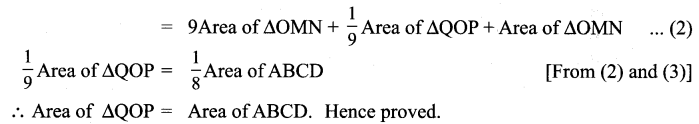## Tamilnadu Samacheer Kalvi 9th Maths Solutions Chapter 4 Geometry Ex 4.2

Question 1.
The angles of a quadrilateral are in the ratio 2 : 4 : 5 : 7. Find all the angles.
Solution:
Let’s call the angles 2x, 4x, 5x, 7x
2x + 4x + 5x + 7x = 360°
18x = 360°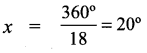A = 2x = 2 × 20° = 40°
B = 4x = 4 × 20° = 80°
C = 5x = 5 × 20° = 100°
D = 7x = 7 × 20° = 140°

Question 2.
In a quadrilateral ABCD, ∠A = 12° and ∠C is the supplementary of ∠A. The other two angles are 2x – 10 and x + 4. Find the value of x and the measure of all the angles.
Solution:
∠A = 72°
∠C = 180° – 72° (∵ Supplementary at ∠A) = 108°
The other two angles are 2x – 10 and x + 4.
2x – 10 + x + 4 + 108° + 12° = 360°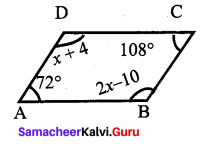3x + 174° = 360°
3x = 360° – 174°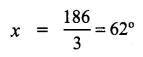∴ ∠A = 72°
∠B = 2x – 10 = 2(62)- 10 = 124 – 10 = 114°
∠C = 108°
∠D = x + 4 = 62 + 4 = 66°

Question 3.
ABCD is a rectangle whose diagonals AC and BD intersect at O. If ∠OAB = 46°, find ∠OBC.
Solution:
∠ABC = 90°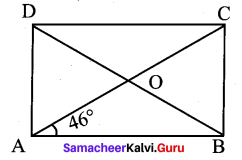∠OAB + ∠OBC = 90°
46° + ∠OAB = 90°
∠OBC = 90° – 46° = 44°

Question 4.
The lengths of the diagonals of a Rhombus are 12 cm and 16 cm. Find the side of the rhombus.
Solution:
Let ABCD be a rhombus with AC and BD as its diagonals.
We know that the diagonals of a rhombus bisect each other at right angles.
Let O be the intersecting point of both the diagonals
Let AC = 16 cm and BD = 12 cm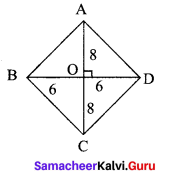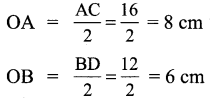use Pythagoras theorem, we have
AB2 = OA2 + OB2
AB2 = 100
∴ AB = 10 cm

Question 5.
Show that the bisectors of angles of a parallelogram form a rectangle.
Solution:
Given ABCD is a parallelogram. Draw the angular bisectors AP, BP, CR and DR of the angles ∠A, ∠B, ∠C and ∠D respectively.
Now to prove : PQRS is a rectangle.
Proof: A rectangle is a parallelogram with one angle 90°.
First we will prove PQRS is a parallelogram.
Now AB || CD and AD is transversal. [ ∴ Interior angles on the same side of transversal are supplementary]
[Opposite sides of a parallelogram are parallel]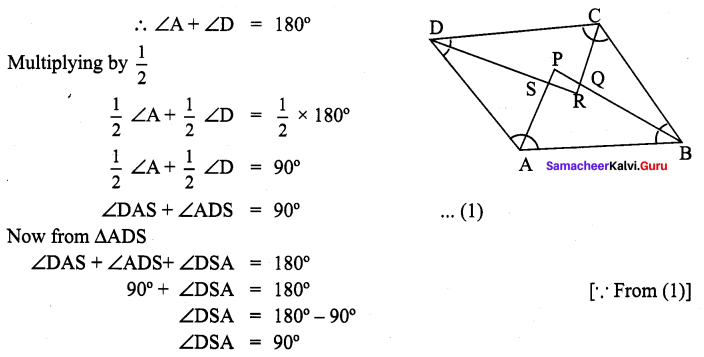Also lines AP and DR intersects
So ∠PSR = ∠DS A
∴ ∠PSR = 90° [∵ Vertically opposite angles]
Similarly we can prove that ∠SPQ = 90°, ∠PQR = 90° and ∠SRQ = 90°
∴ ∠PSR = ∠PQR and ∠SPQ = ∠SRQ
∴ Both pair of opposite angles of PQRS is a parallelogram.
Also ∠PSR = ∠PQR = ∠SPQ = ∠SRQ = 90°
∴ PQRS is a parallelogram with one angle 90°.
∴ PQRS is a rectangle. Hence proved.

Question 6.
If a triangle and a parallelogram lie on the same base and between the same parallels, then prove that the area of the triangle is equal to half of the area of parallelogram.
Solution:
Given: ∆ABE and parallelogram ABCD have the same base and are between the same parallel lines (i.e) l1 || l2.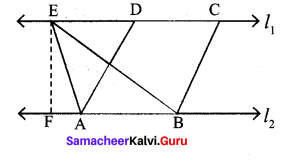Perpendicular distance between l1 and l2 = P (say).
Prove that: area of (∆ABE) = $$\frac{1}{2}$$ × area of the parallelogram ABCD
Proof: Area of ∆ABE = $$\frac{1}{2}$$ × base × height
= $$\frac{1}{2}$$ × AB × (Perpendicular distance between l1
= $$\frac{1}{2}$$ × AB × P ….(1)
Area of parallelogram ABCD = base × height.
∴ Area of parallelogram ABCD = AB × P …. (2)
From (1) and (2),
Area of ∆ABE = $$\frac{1}{2}$$ × Area of parallelogram ABCD.
Hence proved.

Question 7.
Iron rods a, b, c, d, e, and f are making a design in a bridge as shown in the figure. If a || b, c || d, e || f, find the marked angles between
(i) b and c
(ii) d and e
(iii) d and f
(iv) c and f.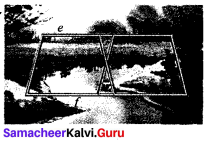Solution:
Since l, m are two parallel lines and PQ, RS, TU, VW are transversal.
Then ∠1 = ∠QOR [vertically opposite angles]
∠1 = 30° [∴ ∠QOR = 30°]
Also, PQ and TU are parallel and m and l are transversal.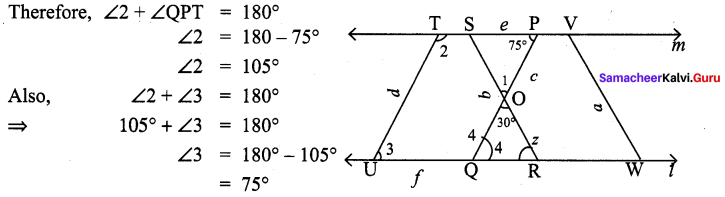Also ∠3 + ∠4 = 180°
⇒ 75° + ∠4 = 180°
∠4 = 180° – 75° = 105°
Hence,
(i) 30°
(ii) 105°
(iii) 75°
(iv) 105°

Question 8.
In the given figure ∠A = 64° , ∠ABC = 58°. If BO and CO are the bisectors of ∠ABC and ∠ACB respectively of ∆ABC, find x° and y°.
Solution:
In ∆ABC, ∠A + ∠B + ∠C = 180°
⇒ 64° + 58° + ∠C = 180°
⇒ 122°+ ∠C = 180°
⇒ ∠C = 180°- 122° = 58°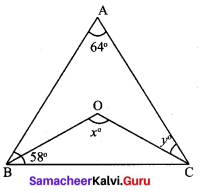Also BO and CO are the bisectors of ∠ABC and ∠ACB respectively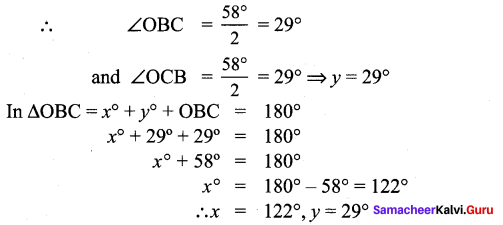Question 9.
In the given Fig. if AB = 2, BC = 6, AE = 6, BF = 8, CE = 7, and CF = 7, compute the ratio of the area of quadrilateral ABDE to the area of ACDF.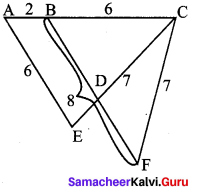Solution:
Given: AB = 2,
BC = 6,
AE = 6,
BF = 8,
CE = 7 and
CF = 7
Consider ∆AEC and ∆BCF,
In ∆AEC,
AC = 8,
AE = 6,
CE = 7
In ∆BCF,
BF = 8,
BC = 6,
CF = 7
∴ ∆AEC ≅ ∆BCF
∴ Area of ∆AEC = Area of ∆BCF
Subtract.area of ∆BDC both sides, we get
Area of ∆AEC – Area of ∆BDC = Area of ∆BCF – Area of ∆BDC
⇒ Area of quadrilateral ABDE = Area of ∆CDF
∴ The required ratio is 1 : 1

Question 10.
In the figure, ABCD is a rectangle and EFGH is a parallelogram. Using the measurements given in the figure, what is the length d of the segment that is perpendicular to $$\overline{\mathbf{H E}}$$ and $$\overline{\mathbf{F G}}$$ ?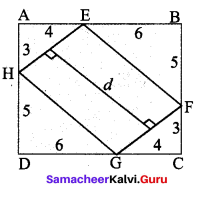Solution:
Area of ABCD = length × breadth.
= DC × BC = 10 × 8 = 80.
Area of ∆AEH = Area of ∆CGF [since they are congruent by RHS rule]
Similarly, Area of ∆BEF = Area of ∆DGH
∴ Area of parallelogram = EFGH = Area of rectangle ABCD – 2(area of ∆AEH) – 2(area of ∆BEF)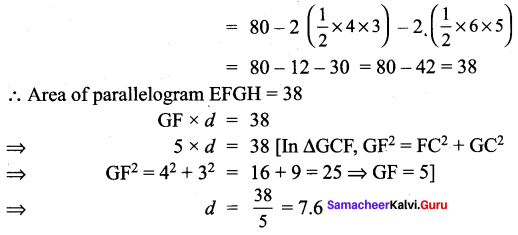Question 11.
In parallelogram ABCD of the accompanying diagram, line DP is drawn bisecting BC at N and meeting AB (extended) at P. From vertex C, line CQ is drawn bisecting side AD at M and meeting AB (extended) at Q. Lines DP and CQ meet at O. Show that the area of triangle QPO is $$\frac{9}{8}$$ of the area of the parallelogram ABCD.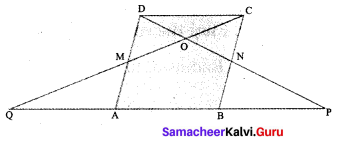Solution: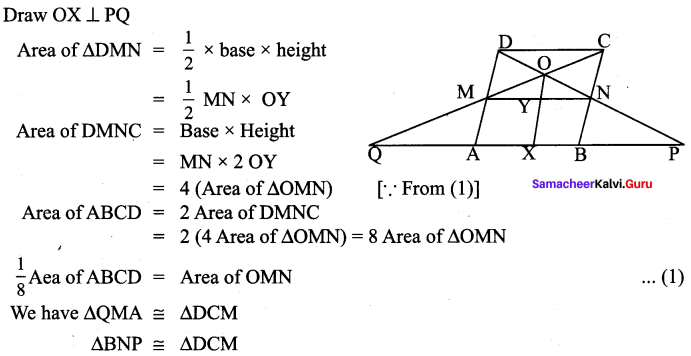Area of ∆QDP = Area of ∆QMA + Area of ∆MNO + Area of MNBS + Area of ∆MAB
= Area of ∆DCM + Area of ∆MNO + Area of MNBA + Area of ∆NDC
= 2Area of ∆OMN + Area of ∆MNO + 4 Area of ∆OMN + 2 Area of ∆OMN